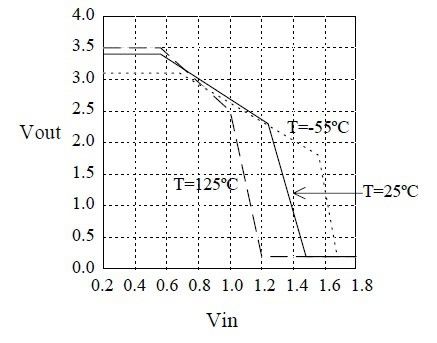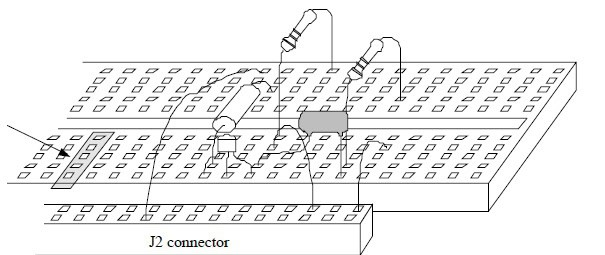# Basic Digital Circuits

## Quiz Questions

 1. In a popular logic family what does TTL stand for? Transistor-Transformer Logic Transistor-Transistor Logic Transformer-Transformer Logic Transformer Testing Logic 2. Gases and liquids exert a force on the sides of whatever container they are in. This force is known as ______________ ? Gravitational force Molecular force Pressure Surface tension 3. The process of fixing problems in a logic circuit is called ___________ ? Correcting Testing Debugging Recreating 4. _____________ is a useful tool for finding problems in digital logic circuits. Logic probe Logic chip Logic wire Logic pen 5. Noise is never present in electrical systems True False 6. Propagation delay is defined as a delay between a change in an input of a logic gate and a resulting change in the output of the gate. True False 7. The effect of propagation delay on circuits is analyzed by means of ___________ ? Delay diagrams Timing diagrams Propagation diagrams Digital diagrams 8. What are the basic types of Delays in Physical systems ______________ ? Pure delay and inertial delay Common delay and pure delay Inertial delay and time delay Common delay and time delay 9. What is the actual range of output voltages from the following diagram?0.2V to 1.2V 1.2V to 1.6V 0.2V to 3.5V 0V to 3.5V 10. The five holes shown by the arrow in the following diagram are electrically connected ?True False